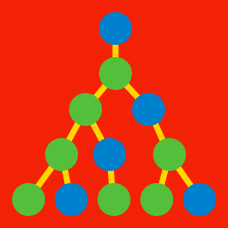Probability

# Linear Recurrence Relations - With Repeated Roots

The sequence $\{x_n\}$ satisfies the relation $\begin{array}{c}&x_1 = 2, &x_2 = 9, &x_{n+2} = 6 x_{n+1} - 9 x_n &(n = 1, 2, 3, \ldots). \end{array}$ What is the value of $x_5?$

The sequence $\{x_n\}$ satisfies the relation $\begin{array}{c}&x_1 = 3, &x_2 = 9, &x_3 = 29, &x_{n+3} = 9 x_{n+2} - 26 x_{n+1} + 24 x_n, \end{array}$ where $n$ is a positive integer. Find the closed-form expression of $x_n.$

The sequence $\{x_n\}$ satisfies the relation $\begin{array}{c}&x_1 = -3, &x_2 = -9, &x_3 = 9, &x_{n+3} = 9 x_{n+2} - 27 x_{n+1} + 27 x_n, \end{array}$ where $n$ is a positive integer. Find the closed-form expression of $x_n.$

The sequence $\{x_n\}$ satisfies the relation $\begin{array}{c}&x_1 = -7, &x_2 = 0, &x_3 = 6, &x_{n+3} = 2 x_{n+2} + 5 x_{n+1} - 6 x_n, \end{array}$ where $n$ is a positive integer. What is the value of $x_6?$

The sequence $\{x_n\}$ satisfies the relation $\begin{array}{c}&x_1 = 0, &x_2 = 1, &x_{n+2} + 2 x_{n+1} + x_n = 0 &(n = 1, 2, 3, \ldots). \end{array}$ If $x_n$ can be expressed as $a r^n + b n r^n,$ what is the value of $\frac{b}{a}?$

×